# Accelerated Failure Time Models with ciTools

#### 12 June, 2020

library(tidyverse)
library(knitr)
library(ciTools)
library(here)
set.seed(20180925)

Disclaimer: ciTools makes three assumptions about your model:

1 - no missing data in the “newdata” matrix, tb.

2 - distribution is one of weibull, lognormal, loglogistic, or exponential.

3 - regression model is unweighted, and without random effects.

The purpose of this vignette is to introduce and discuss new ciTools capabilities for handling accelerated failure time (AFT) models. Some of the new AFT methods in ciTools are more informative than methods for previous models, and will inform future development decisions that will be made in ciTools. In particular, ciTools now supports intervals for estimated survival time probabilities and quantiles for a range of common AFTs.

## The Accelerated Failure Time Model

The accelerated failure time model is, like a generalized linear model (GLM), an extension of the standard linear model that accounts for specific types of data and non-linearity. AFTs constitute and important class of models as they can handle censored, highly skewed data – exactly the type of data one would expect to collect when analyzing the failure times of a machine, or the survival times of a group of patients under study.

AFTs are special in the field of survival/reliability analysis in that they are fully parametric models. This provides power to do certain inferences such as the estimation of tail probabilities that would be difficult in a non or semi-parametric framework. The trade-off made is that a specific distribution for survival times must be assumed, and this assumption may be incorrect.

The structure of accelerated failure time models is as follows. We observe a vector of survival times (failure times, in the reliability literature) $$T$$ given a data matrix $$X$$. We assume the $$\log$$ of the survival times is affected linearly by the covariates of $$X$$. Because $$T$$ is non-negative, we model the effect of the linear predictor $$X\beta$$ on $$\log(T)$$. The model is

$F(T|X) = F \left( \frac{\log(T) - X\beta}{\sigma} \right).$

$$F$$ denotes a vectorized, standard distribution function; $$X\beta$$ is called the linear predictor; and $$\sigma$$ is called the scale parameter. $$S(T|X)$$ is called he survivor function; the probability that a unit will fail after time $$T$$. $$S(T|X) = 1 - F(T|X)$$. Thus the AFT model is a family of log-linear models. Examples of $$F$$ that are common are the standard normal, standard logistic, and standard smallest extreme value distribution functions (Meeker and Escobar, Ch. 4). We can more clearly write the model as

$\log(T) = X\beta + \sigma \varepsilon,$

where $$\varepsilon \sim F$$ to make the linear effect of $$\beta$$ on $$\log(T)$$ a bit more apparent.

Like generalized linear models, survival models are fit through a maximum likelihood procedure. This is most useful in that it allows a practitioner to specify censored data in the statistical model. That AFTs are fully parametric and may account for data censoring were primary reasons for adding them to ciTools. We assume that AFTs are fit in R with the survreg function from the survival library.

### Examples of AFTs

Four examples of AFT models are presented, which are covered completely by ciTools. This list of AFT models is not exhaustive, as other models are available. See the flexsurv package, for example. Models in the flexsurv package do not presently receive treatment by ciTools.

1. Lognormal: Let $$\varepsilon \sim N(0,1)$$. Then $$\log(T) = X\beta + \sigma \varepsilon$$ and $$T$$ is said to be lognormal with parameters $$X\beta$$ and $$\sigma$$. Confidence intervals for the following parameters are available in ciTools.

$E(T|X) = \exp(X \beta + \frac{\sigma^2}{2}) \qquad (\text{expected time to failure})$

$\text{median}(T|X) = \exp(X\beta) \qquad (\text{median time to failure})$

$S(T|X) = 1 - \Phi \left( \frac{\log(T) - X\beta}{\sigma} \right) \qquad \Phi \sim \text{std. Normal CDF}$

$F^{-1}_p(T|X) = \exp(X\beta + \Phi^{-1}(p) \sigma) \qquad (\text{level p quantile of failure time distribution})$

1. Weibull: Let $$\varepsilon$$ possess smallest extreme value distribution. We write $$\varepsilon \sim SEV$$ with $$F_{SEV}(\varepsilon) = 1-\exp(-\exp(\varepsilon))$$. If $$\log(T) = X\beta + \sigma\varepsilon$$, then $$T$$ is weibull distributed with scale parameter $$\sigma$$ and location parameter $$\exp(X\beta)$$ in the location-scale parameterization used in the survival package. This parameterization differs from the one used in {p/d/q/r}weibull, see help(survreg) for details.

$E(T|X) = \exp(X\beta)\Gamma(1 + \sigma)$

$\text{median}(T|X) = \exp(X\beta + F^{-1}_{SEV}(0.5) \sigma) = \exp(X\beta)(\log(2))^{\sigma}$

$F^{-1}_p(T|X) = \exp(X\beta + F^{-1}_{SEV}(p) \sigma) = \exp(X\beta)(-\log(1-p))^{\sigma}$

$S(T|X) = \exp(-\exp(z)), \qquad z = \frac{\log(T) - X\beta}{\sigma}$

1. Exponential: Like the weibull distribution, except scale parameter $$\sigma$$ is fixed to $$1$$.

$E(T|X) = \exp(X\beta)$

$\text{median}(T|X) = \exp(X\beta + F^{-1}_{SEV}(0.5)) = \exp(X\beta)\log(2)$

$F^{-1}_p(T|X) = \exp(X\beta + F^{-1}_{SEV}(p)) = \exp(X\beta)(-\log(1-p))$

$S(T|X) = \exp(-\exp(z)), \qquad z = \log(T) - X\beta$

1. Loglogistic: Let $$\varepsilon \sim \text{Logistic}$$. That is, $$F(\varepsilon) = \frac{\exp(\varepsilon)}{1 + \exp(\varepsilon)}$$, the standard logistic distribution. Then $$\log(T) = X\beta + \sigma\varepsilon$$, and $$T$$ is loglogistic distributed with scale parameter $$\sigma$$ and location parameter $$X\beta$$.

$E(T|X) = \exp(X\beta)\Gamma(1 + \sigma)\Gamma(1 - \sigma)$

$\text{median}(T|X) = \exp(X\beta)$

$F^{-1}_p(T|X) = \exp(X\beta + \sigma F^{-1}_{Logistic}(p))$

$S(T|X) = 1 - F_{Logistic} \left( \frac{\log(T) - X\beta}{\sigma} \right)$

Note that the median of each conditional failure time distribution is technically the level $$p=0.5$$ quantile of that same distribution. For this reason, confidence intervals for medians are calculated with add_quantile().

## AFT Uncertainty Intervals

In the analysis of AFT models, statisticians have several options for making predictions. predict.survreg for example, allows one to predict the median failure time, or any other quantile. These predictions have the same units as the original time scale (time to death or failure). Additionally, predict.survreg can also output the corresponding value of the linear predictor for a given point in the factor space.

ciTools hopes to clarify survival times prediction for AFT models by relegating prediction of the expected (mean) survival time to add_ci.survreg, and prediction of the median (or any quantile) of the survival time distribution to add_quantile.survreg. Thus add_ci.survreg is in line with other add_ci S3 methods provided by ciTools by only providing confidence intervals for the expected response conditioned on the predictors.

There are three popular methods for forming confidence intervals in this case: parametrically, using either the (1) delta method or (2) likelihood ratios, or (3) through a bootstrap resampling procedure. In ciTools, we generally favor parametric methods, except where it makes sense to include bootstrap methods as options, as is the case with many mixed effects models, where bootstrap methods are seen as less controversial than parametric interval methods.

We have studied these three techniques for interval estimation, and found that the delta method offers the best combination of speed and accuracy for users. Therefore, the delta method is used as the basis of all interval estimation procedures in ciTools for AFT models. This is at odds with the recommendations of Meeker and Escobar in favor of likelihood based intervals, however we implement delta method based intervals as they are much easier to write for multivariate models and do not suffer from any convergence issues. Compared to bootstrap intervals, delta method intervals are faster and have similar probability coverage in many scenarios.

## Example.

Data are collected on the failure times of a new spring installed in a car. The spring can be mounted in two types of cars, an SUV or a sedan. An additional variable, ambient temperature was also recorded. Experimental vehicles were fitted with the new springs, and the vehicles are placed into an observational study. Vehicles with the new springs were driven, and the failure times of the springs were noted until the conclusion of the test time. The test concluded after $$2000$$ cumulative hours of testing. At this time, all surviving springs at marked as right censored at $$t=2000$$. All data are notional.

The time variable indicates of the number of hours driven before a spring failure is observed. If failure = 1, a spring failure is observed at the indicated time.

kable(head(dat))
temp car time failure
40.00000 suv 5.6414974 1
41.22449 sedan 2.5287376 1
42.44898 suv 44.3712587 1
43.67347 sedan 12.1143060 1
44.89796 suv 0.0344103 1
46.12245 sedan 198.6757464 1
ggplot(dat, aes(x = temp, y = time)) +
geom_point(aes(color = factor(failure)))+
ggtitle("Censored obs. in red") +
theme_bw()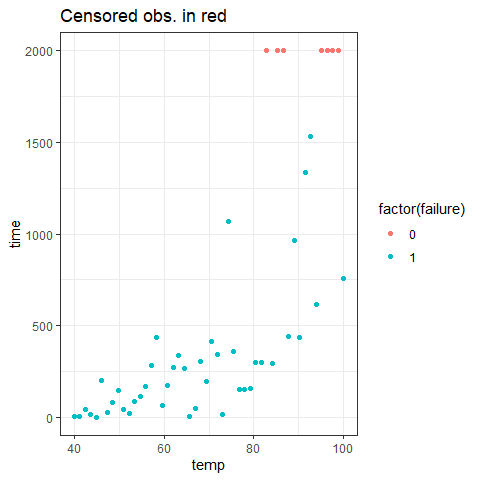Seven of the observations are censored at $$t = 2000$$. This means we assume those $$7$$ springs would eventually fail at some later point in time had we chosen to run the study for a longer period of time. We fit a weibull model to the data. By default, Surv will infer (correctly, in this case) that our observations are right censored at $$t=2000$$. Check the documentation of Surv for how to coordinate a different censoring regime – survreg is very flexible in the types of censoring allowed (another advantage of AFT models). Other distributions (exponential, lognormal, loglogistic) are available in survreg for parametric analysis, and receive treatment in ciTools, but we stick the weibull model for the example.

(fit <- survreg(Surv(time, failure) ~ temp + car, data = dat)) ## weibull dist is default
## Call:
## survreg(formula = Surv(time, failure) ~ temp + car, data = dat)
##
## Coefficients:
## (Intercept)        temp      carsuv
##  0.31303047  0.08126381 -0.25327482
##
## Scale= 1.019839
##
## Loglik(model)= -283.3   Loglik(intercept only)= -307.8
##  Chisq= 48.98 on 2 degrees of freedom, p= 2.32e-11
## n= 50

The output of survreg indicates that the model on the whole is significantly better than one which does not include any covariates. Maximum likelihood estimates of coefficients are displayed as well. We can analyze the model graphically with the help of ciTools. The summary function can be called on fit to show some additional information about the model coefficients. We calculate confidence and prediction intervals, and append them to the original data set.

with_ints <- ciTools::add_ci(dat,fit, names = c("lcb", "ucb")) %>%
ciTools::add_pi(fit, names = c("lpb", "upb"))
kable(head(with_ints))
temp car time failure mean_pred lcb ucb median_pred lpb upb
40.00000 suv 5.6414974 1 27.62779 15.72767 48.53195 18.85020 0.6447620 103.7026
41.22449 sedan 2.5287376 1 39.31490 23.04415 67.07392 26.82422 0.9175093 147.5709
42.44898 suv 44.3712587 1 33.71138 19.77579 57.46708 23.00098 0.7867375 126.5378
43.67347 sedan 12.1143060 1 47.97198 28.92116 79.57187 32.73086 1.1195433 180.0658
44.89796 suv 0.0344103 1 41.13457 24.83171 68.14080 28.06576 0.9599757 154.4012
46.12245 sedan 198.6757464 1 58.53533 36.23626 94.55678 39.93814 1.3660649 219.7160

The output of ciTools’s functions is always the inputted data with the requested statistics attached. The inputted data can be original data or a data frame of new observations. For this model fit, add_ci calculates conditional means (denoted mean_pred in the data frame) and add_pi calculates conditional medians (median_pred in the data frame).

ggplot(with_ints, aes(x = temp, y = time)) +
geom_point(aes(color = car)) +
facet_wrap(~car)+
theme_bw() +
ggtitle("Model fit with 95% CIs and PIs",
"solid line = mean, dotted line = median") +
geom_line(aes(y = mean_pred), linetype = 1) +
geom_line(aes(y = median_pred), linetype = 2) +
geom_ribbon(aes(ymin = lcb, ymax = ucb), alpha = 0.5) +
geom_ribbon(aes(ymin = lpb, ymax = upb), alpha = 0.1)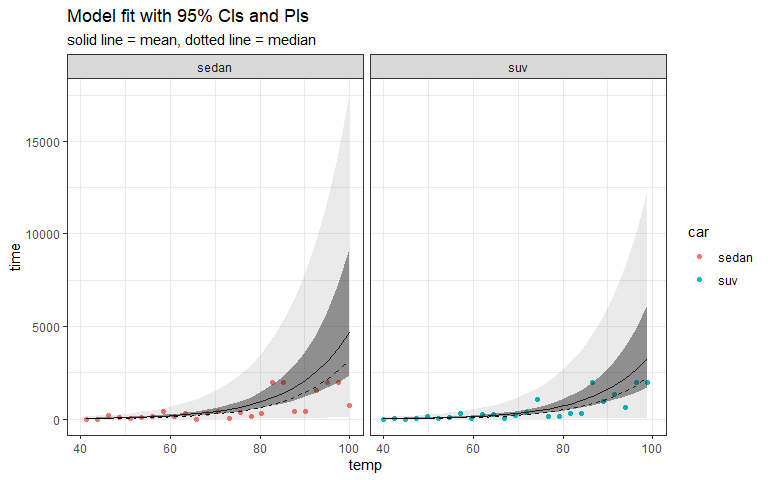probs <- ciTools::add_probs(dat, fit, q = 500,
name = c("prob", "lcb", "ucb"),
comparison = ">")

We can calculate the estimated survival probabilities as well. Below, we calculate the probability of a spring failing after $$t = 500$$ (alternatively, the probability of a spring not failing before $$t = 500$$). This is not a new feature to ciTools, what’s special to survreg methods is that ciTools will additionally compute confidence intervals for the estimated conditional survival probabilities.

ggplot(probs, aes(x = temp, y = prob)) +
ggtitle("Estimated prob. of avg. spring lasting longer than 500 hrs.") +
ylim(c(0,1)) +
facet_wrap(~car)+
theme_bw() +
geom_line(aes(y = prob)) +
geom_ribbon(aes(ymin = lcb, ymax = ucb), alpha = 0.5)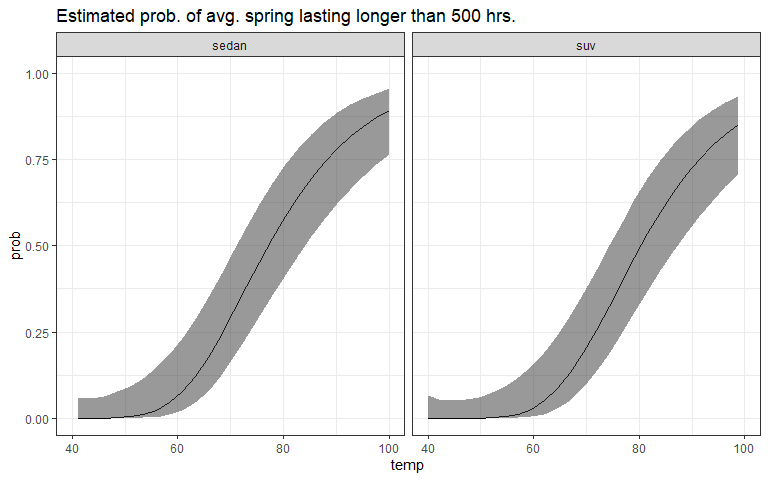quants <- ciTools::add_quantile(dat, fit, p = 0.90,
name = c("quant", "lcb", "ucb"))

Furthermore, we can calculate quantiles of the distribution of failure times given the covariates in dat. Again, the special sauce for AFT models comes when ciTools also tacks on confidence intervals for the estimated quantiles. Here, we show the estimated $$0.90$$ quantile, conditioned on the covariate information, with confidence intervals. One may use add_quantile to calculate the median failure time, or any other quantile.

ggplot(quants, aes(x = temp, y = time)) +
geom_point(aes(color = car)) +
ggtitle("Estimated 90th percentile of condtional failure distribution, with CI") +
facet_wrap(~car)+
theme_bw() +
geom_line(aes(y = quant)) +
geom_ribbon(aes(ymin = lcb, ymax = ucb), alpha = 0.5)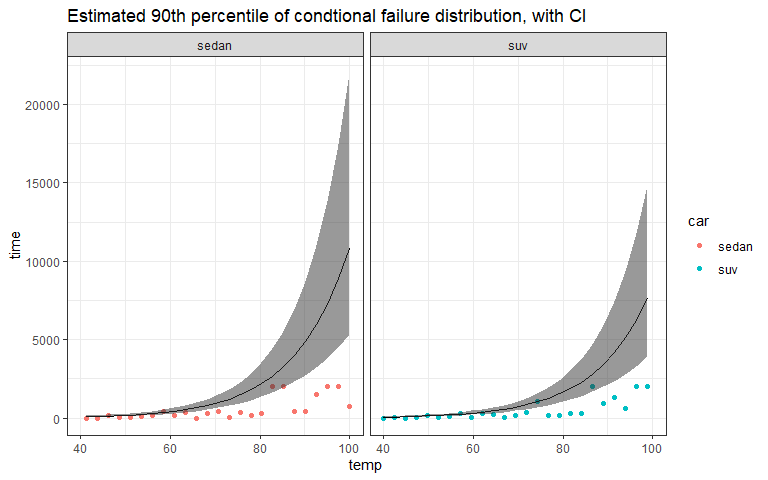## The Delta Method for Regression Models

Here are the mathematical details for calculating the above confidence intervals. For AFT models, we calculate confidence intervals with the delta method (Prediction intervals are calculated using different methods, discussed later).

Let $$\boldsymbol{\theta} = (\beta_0, \beta_1, \ldots, \beta_p, \sigma)$$ be the vector of maximum likelihood parameter estimates for the statistical model. We wish to form confidence intervals for continuous and twice differentiable functions of $$\boldsymbol{\theta}$$, say $$\mathbf{g}(\boldsymbol{\theta})$$. Because $$\hat{\boldsymbol{\theta}}_{ML}$$ is a maximum likelihood estimator of $$\boldsymbol{\theta}$$, $$\mathbf{g}(\hat{\boldsymbol{\theta}_{ML}})$$ is a maximum likelihood estimator of $$\mathbf{g}(\boldsymbol{\theta})$$. In large samples, $$\mathbf{g}(\hat{\boldsymbol{\theta}})$$ is approximately Normally distributed with mean $$\mathbf{g}(\boldsymbol{\theta})$$ and variance-covariance matrix

$\Sigma_{\hat{g}} = \left[ \frac{\partial \mathbf{g} (\boldsymbol{\theta})}{\partial \boldsymbol{\theta}}\right]^T \Sigma_{\hat{\boldsymbol{\theta}}} \left[ \frac{\partial \mathbf{g} (\boldsymbol{\theta})}{\partial \boldsymbol{\theta}}\right].$

This approximation is based on the assumption that $$\mathbf{g}({\hat{\boldsymbol{\theta}}})$$ is linear in $$\hat{\boldsymbol{\theta}}$$ in a region near $$\boldsymbol{\theta}$$. The larger the sample, the better, because the variation in $$\hat{\boldsymbol{\theta}}$$ decreases with sample size and thus the region over which $$\hat{\boldsymbol{\theta}}$$ varies is correspondingly smaller. If the region is small enough, the approximation is adequate.

Mathematically, the delta method is a statistical rebranding of the a Taylor series expansion for $$\mathrm{Var}[\mathbf{g}(\hat{\boldsymbol{\theta}})]$$ . We will use the delta method to form confidence intervals for functions of $$\boldsymbol{\theta}$$: expected values, quantiles, and survivor functions.

## Expected Values

Because it is somewhat easier to explain confidence intervals for the mean if we have a particular model in mind, suppose for the moment that we fit a Weibull AFT model. The expected survival time, $$\mathrm{E}[T|X]$$, written as a function of $$\boldsymbol{\theta}$$, is $$\mathbf{g}(\boldsymbol{\theta}) = \exp(X\beta)\Gamma(1 + \sigma)$$. We form a confidence interval for this mean survival time based on the estimated regression coefficients ($$\hat{\boldsymbol{\beta}}$$, and $$\hat{\sigma}$$) from survreg. Due to some quirks in numerical optimization, it is often advantageous to reparameterize the scale parameter as $$\delta = \log(\sigma)$$ in the model. Let $$x$$ denote a point in the factor space at which we wish to calculate the expected failure time. The relevant derivatives are

$\frac{\partial \mathbf{g} (\boldsymbol{\theta})}{\partial \boldsymbol{\beta}} = \exp(x^T \boldsymbol{\beta}) x^T \Gamma(1 + \exp(\delta)) ,\qquad i = 0, \ldots, p,$ and $\frac{\partial \mathbf{g} (\boldsymbol{\theta})}{\partial \delta} = \exp(x^T \boldsymbol{\beta}) \Gamma(1 + \exp(\delta)) \psi(1 + \exp(\delta)) \exp(\delta),$

where $$\psi(\cdot)$$ denotes the digamma function. Let $$\frac{\partial\mathbf{g}(\boldsymbol{\theta})}{\partial \boldsymbol{\theta}} = \left(\frac{\partial \mathbf{g}(\boldsymbol{\theta})}{\partial \beta_0}, \frac{\partial \mathbf{g}(\boldsymbol{\theta})}{\partial \beta_1}, \frac{\partial \mathbf{g}(\boldsymbol{\theta})}{\partial \beta_2}, \ldots, \frac{\partial \mathbf{g}(\boldsymbol{\theta})}{\partial \beta_p}, \frac{\partial \mathbf{g}(\boldsymbol{\theta})}{\partial \delta}\right)^T$$.

The standard error of the expected survival time estimate is

$\mathrm{s.e.}(\mathbf{g} (\hat{\boldsymbol{\theta}})) = \sqrt{\left[ \frac{\partial \mathbf{g} (\boldsymbol{\theta})}{\partial \boldsymbol{\theta}}\right]^T \Sigma_{\hat{\boldsymbol{\theta}}} \left[ \frac{\partial \mathbf{g} (\boldsymbol{\theta})}{\partial \boldsymbol{\theta}}\right]}.$

An approximate $$100\times(1 - \alpha)\%$$ confidence interval for $$\mathbf{g}(\boldsymbol{\theta}) = \mathrm{E}[T]$$ based on the large sample standard Normal approximation of $$Z_{\log(\mathbf{g}(\hat{\boldsymbol{\theta}}))} = \frac{\log(\mathbf{g}(\hat{\boldsymbol{\theta}})) - \log(\mathbf{g}(\boldsymbol{\theta}))}{s.e.(\mathbf{g} (\hat{\boldsymbol{\theta}}))}$$ is

$\left[\mathrm{lower}, \mathrm{upper} \right] = \left[\mathbf{g}(\hat{\boldsymbol{\theta}})/w, \mathbf{g}(\hat{\boldsymbol{\theta}}) \times w \right],$

where $$w = \exp(z_{1-\alpha/2} \times \mathrm{s.e.}(\mathbf{g} (\hat{\boldsymbol{\theta}})) / \mathbf{g}(\hat{\boldsymbol{\theta}}))$$.

Confidence intervals for the expected response of other AFT models may be calculated similarly, except that the function $$\mathbf{g}$$ depends on the response distribution. For other functions of $$\boldsymbol{\theta}$$ such as the survivor function or response quantile, we apply the delta method as well.

### Simulation Setup

A simulation was conducted to investigate the performance of uncertainty intervals for AFT models. We varied sample size, distribution, and the proportion of observations censored. In all simulations, a simple AFT models with one predictor was used. A time censoring mechanism was assumed and set at one of three levels: no censoring, mild censoring (30% of observations censored) or moderate censoring (50% of observations censored).

The number of simulations for each combination of distribution and censoring was $$10,000$$ (for the confidence intervals) or $$5000$$ (for prediction intervals, survival probabilities, and quantiles).

### Simulation for expected value CIs

We produce graphs of the performance of the delta method for confidence intervals on the expected survival time. Below we show the observed coverage probability as sample size, distribution, and censoring proportion vary. The nominal coverage probability is set at 90% for all simulations.

We observe acceptable performance from the delta method in this case. Larger amounts of censoring (30% and 50%) produce mostly lower coverage probabilities for all distributions except the exponential distribution. The worst case scenario appears to be small sample Lognormal fits with moderate censoring. In this case, there is a near 10% gap between the nominal and observed coverage probabilities.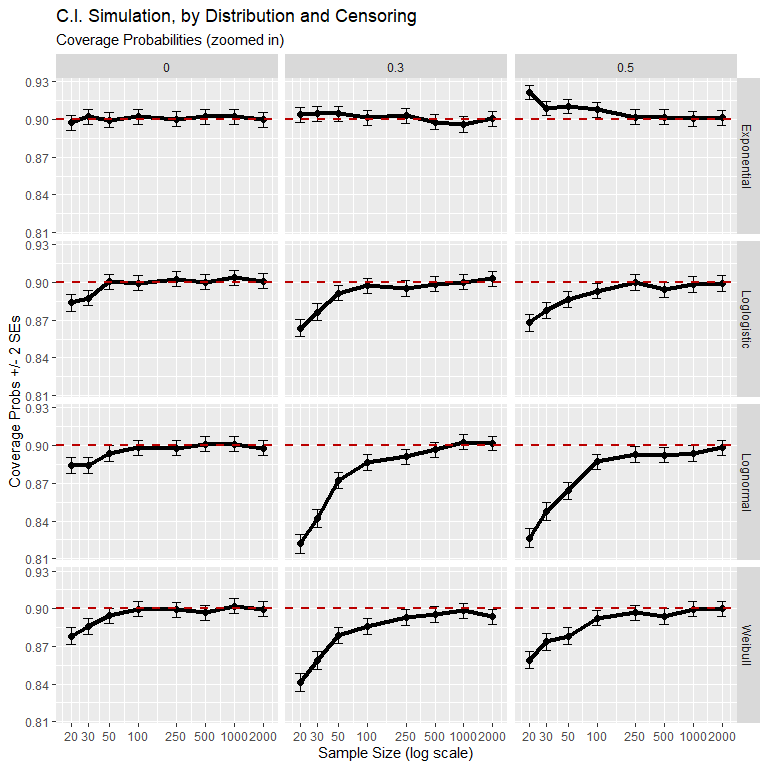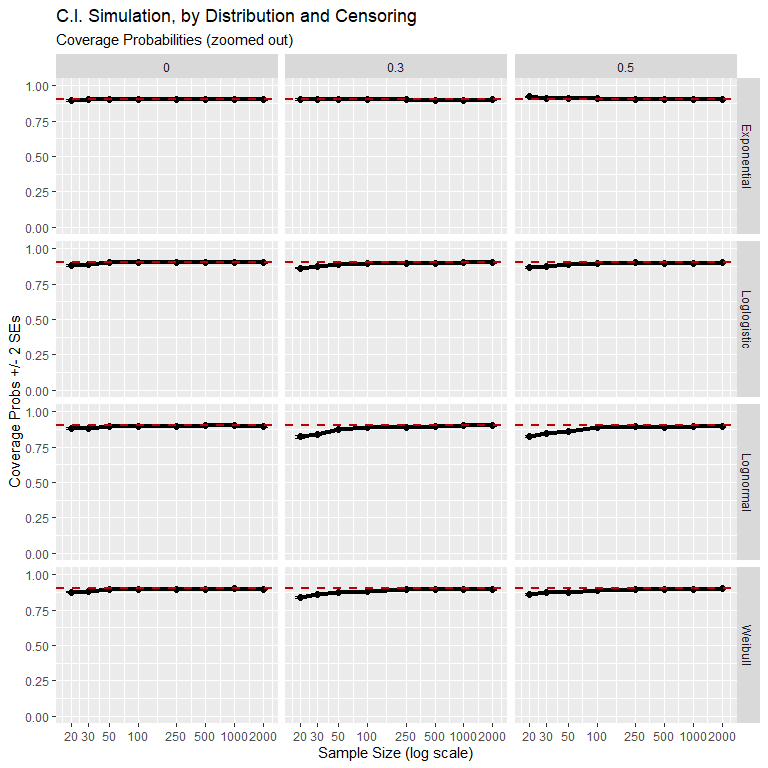Interval widths generally shrink to zero, which is expected. In one case, Lognormal fits with sample size 20 and 50% censoring, the interval widths are too large. This is due to too many unconverged maximum likelihood estimates.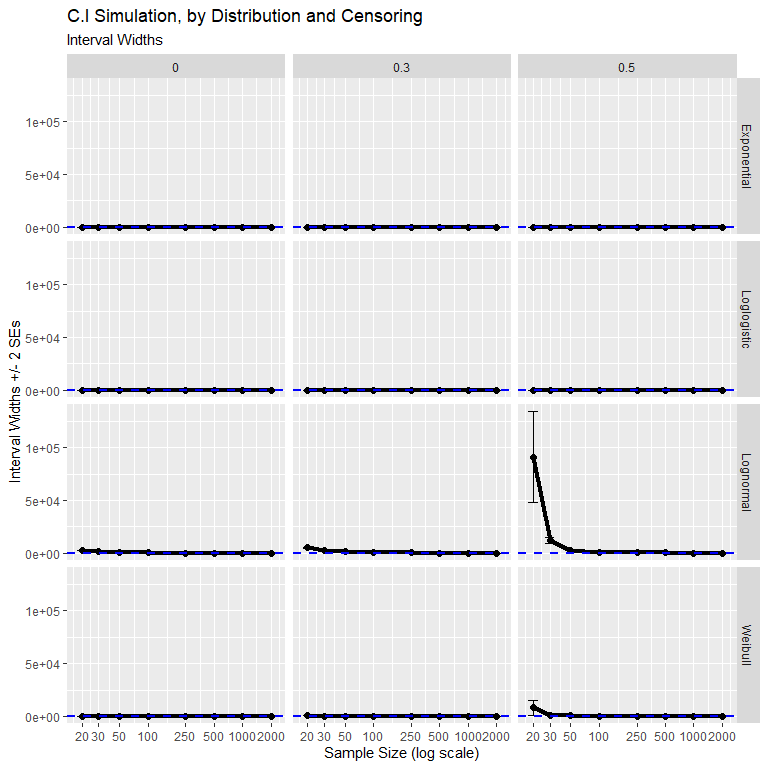## Survivor Function

Calculating confidence intervals for estimated probabilities requires a bit more care to ensure that the confidence bounds lie in the (0,1) interval. Because the mathematics of the confidence intervals for the survivor function depend less on the actual distribution, we won’t focus on the Weibull model, and will treat all AFT models at once. The predicted probability of survival at time $$T$$, $$\hat{S}(T|X)$$, written as a function of $$\boldsymbol{\theta}$$, is $$\mathbf{g}(\boldsymbol{\theta}) = 1 - \Phi \left( \frac{\log(T) - X\boldsymbol{\beta}}{\sigma}\right)$$. Similar to the previous example, we form a confidence interval for this probability of survival based on the estimated regression coefficients ($$\hat{\boldsymbol{\beta}}$$ and $$\hat{\sigma}$$) from survreg. The maximum likelihood estimator of the survivor function is $$\hat{S}(T|X)_{ML} = \Phi \left( \frac{\log(T) - X\hat{\beta}}{\hat{\sigma}}\right)$$. The relevant derivatives are

$\frac{\partial \mathbf{g} (\boldsymbol{\theta})}{\partial \beta} = f \left(\frac{\log(T) - x^T \boldsymbol{\beta}}{\sigma}\right) \times \left(\frac{-x^T}{\sigma}\right),\qquad i = 0, \ldots, n,$

and

$\frac{\partial \mathbf{g} (\boldsymbol{\theta})}{\partial \delta} = f \left(\frac{\log(T) - x^T \boldsymbol{\beta}}{\sigma}\right) \times \left(\frac{x^T \boldsymbol{\beta} - \log(T)}{\sigma} \right),$

where $$f(\cdot)$$ denotes the probability density function corresponding to $$\Psi$$, and $$x_i$$ is a new observation\$. Let $$\frac{\partial\mathbf{g}(\boldsymbol{\theta})}{\partial \boldsymbol{\theta}} = \left(\frac{\partial \mathbf{g}(\boldsymbol{\theta})}{\partial \beta_0}, \frac{\partial \mathbf{g}(\boldsymbol{\theta})}{\partial \beta_1}, \frac{\partial \mathbf{g}(\boldsymbol{\theta})}{\partial \beta_2}, \ldots, \frac{\partial \mathbf{g}(\boldsymbol{\theta})}{\partial \beta_p}, \frac{\partial \mathbf{g}(\boldsymbol{\theta})}{\partial \delta}\right)^T$$.

Due to the delta method, the mathematical form of the standard errors of the estimated survival probability is as it was in the previous example:

$\mathrm{s.e.}(\mathbf{g} (\hat{\boldsymbol{\theta}})) = \sqrt{\left[ \frac{\partial \mathbf{g} (\boldsymbol{\theta})}{\partial \boldsymbol{\theta}}\right]^T \Sigma_{\hat{\boldsymbol{\theta}}} \left[ \frac{\partial \mathbf{g} (\boldsymbol{\theta})}{\partial \boldsymbol{\theta}}\right]}$

The obvious confidence interval based on the statistic $$\frac{\hat{S} - S}{\hat{s.e.}(\hat{S})}$$ could be potentially a very poor fit approximation. The approximation could be poor due to a small to moderate number of failures in the data (Meeker and Escobar, p. 190) or because the bounds of the confidence interval could far exceed the interval $$[0,1]$$. The chosen solution is to apply a transformation $$u(\cdot)$$ such that $$\frac{u(\hat{S}) - u(S)}{\hat{s.e.}(\hat{u})}$$ is closer in distribution to a standard Normal distribution. A transformation that achieves this is the logistic transform.

$u(\hat{S}) = \log \left( \frac{\hat{S}}{1 - \hat{S}} \right)$

First, we find a confidence interval for $$u(\hat{S})$$, then transform the endpoints of the interval to the $$[0,1]$$ through the inverse logistic function to find a confidence interval for $$\hat{S}$$.

$\left[\mathrm{lower}, \mathrm{upper} \right] = \left[ \frac{\hat{S}}{\hat{S} + (1 - \hat{S}) \times w}, \frac{\hat{S}}{\hat{S} + (1 - \hat{S})/w} \right]$

where $$w = \exp \left( \frac{z_{1 - \alpha/2} \hat{s.e.} (\hat{S}) } {\hat{S}(1 - \hat{S})}\right)$$.

### Simulations for Survivor Function

Plots below show the performance of the delta method for uncertainty intervals of the survivor function. Again we compare the observed coverage probability with the 90% nominal probability. In contrast to intervals for the mean, censoring appears to produce overly conservative intervals. This is particularly clear in the case of the Weibull distribution.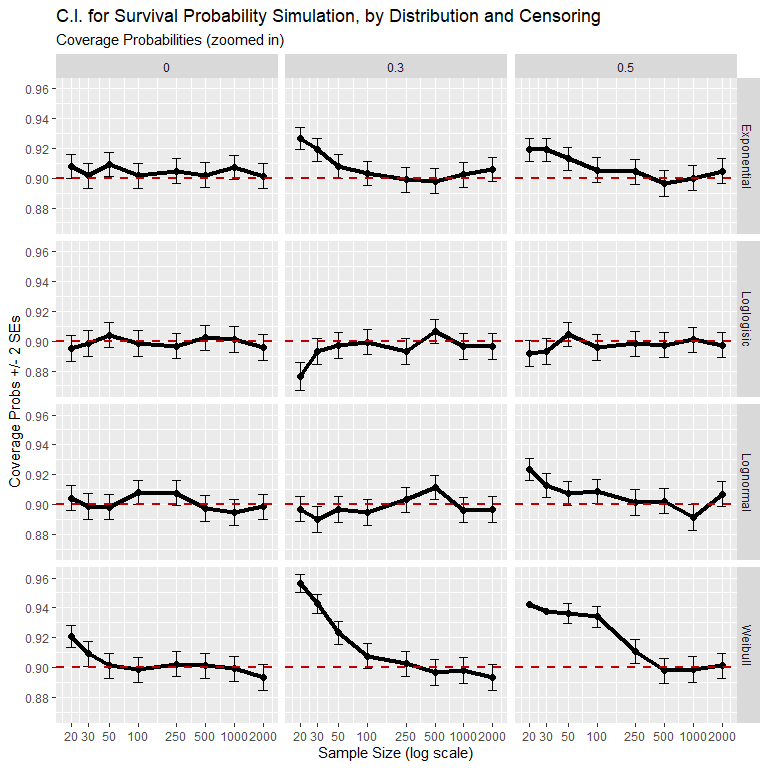However on the (0,1) probability scale, we find acceptable performance.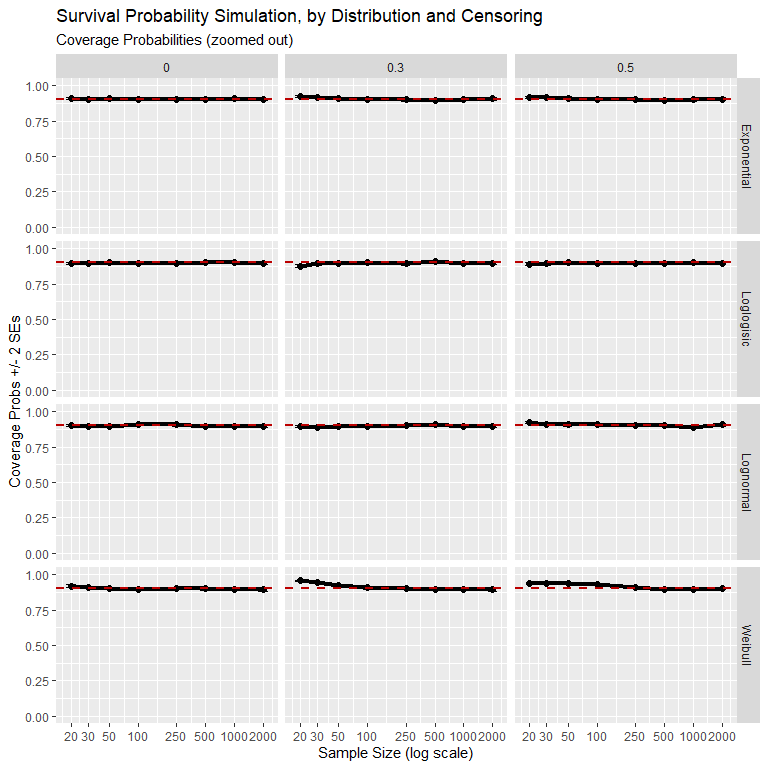Intervals widths go to zero as sample size increases.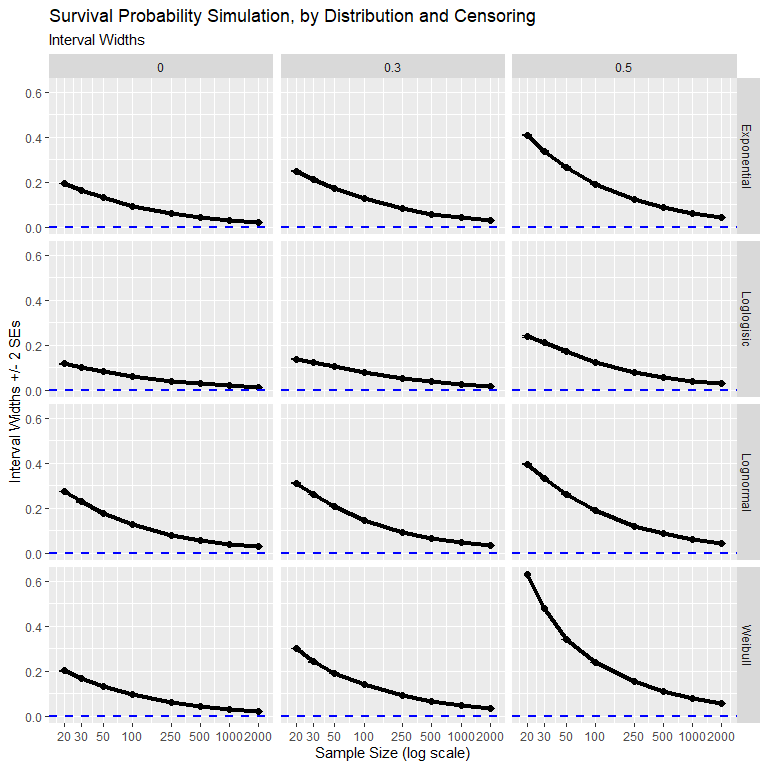## Prediction Intervals

We have not yet discussed prediction intervals, but ciTools also has methods for creating two different types of prediction intervals. The first type is the “naive” method of Meeker and Escobar. The naive method simply forms a prediction interval based on $$\alpha/2$$ and $$1-\alpha/2$$ quantiles of the estimated conditional distribution. The method is naive in the sense that it does not account for uncertainty in the estimates of the parameters $$\boldsymbol{\beta}$$ and $$\sigma$$. This method is simple and works reasonably well in the absence of censoring, as displayed in the plots below.

A slightly better method is included in ciTools, which we call the simulation method. We generate prediction intervals for the next failure time by a parametric bootstrap. This simulation method assumes a multivariate normal distribution for the model coefficients (excluding the scale parameter) and generates new responses that account for this uncertainty in the model coefficients, $$\boldsymbol{\beta}$$. This should make the bootstrap method slightly better than the naive method in practice, though a bit more computationally expensive. This is essentially what we have done to produce prediction intervals for GLMs as well, just applied to the new class of AFT models.

From the plot below, it’s pretty clear that the bootstrap method outperforms the naive method. The difference is most stark when there is a moderate amount of censoring.

Further improvements beyond the naive and simulations methods may be made. These techniques are detailed in Meeker and Escobar (Chapter 12), but have yet to be implemented in ciTools.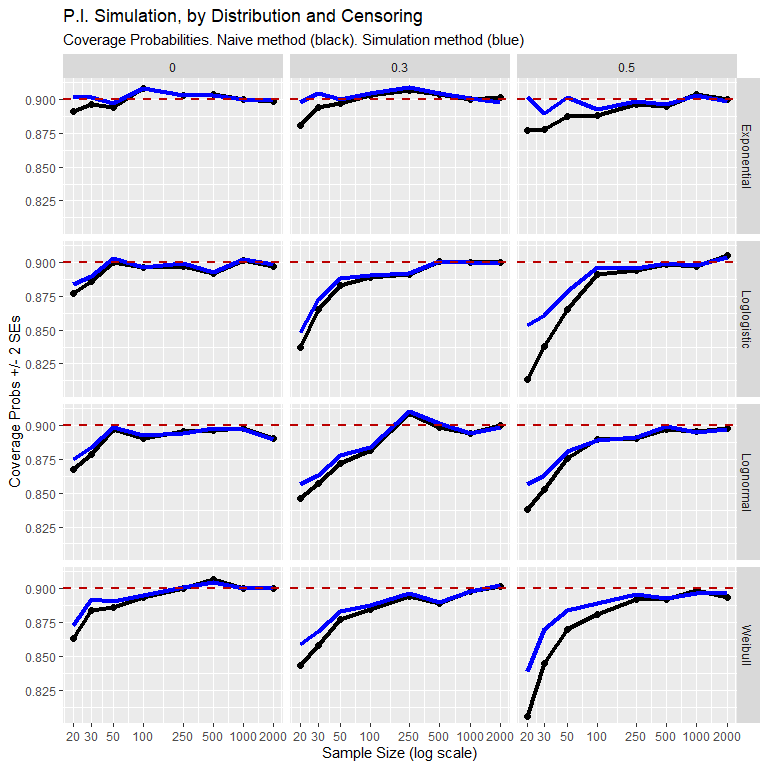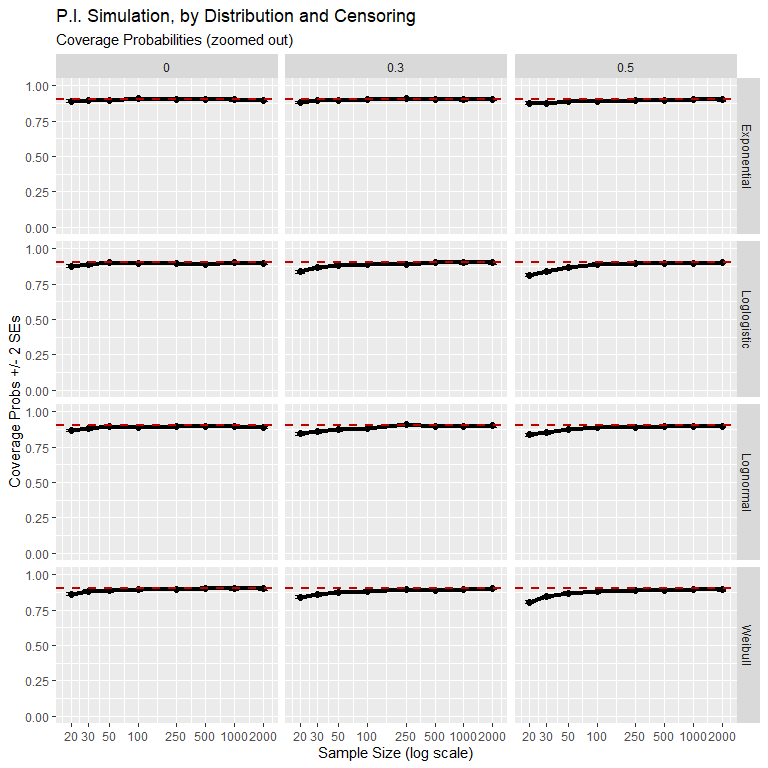Unlike confidence intervals, interval widths of prediction intervals should not shrink to zero as sample size is increased. Instead we should observe interval widths converge to a constant.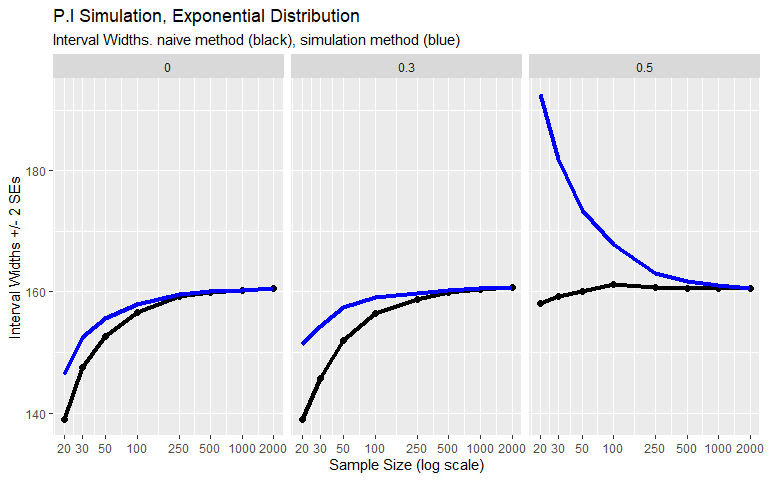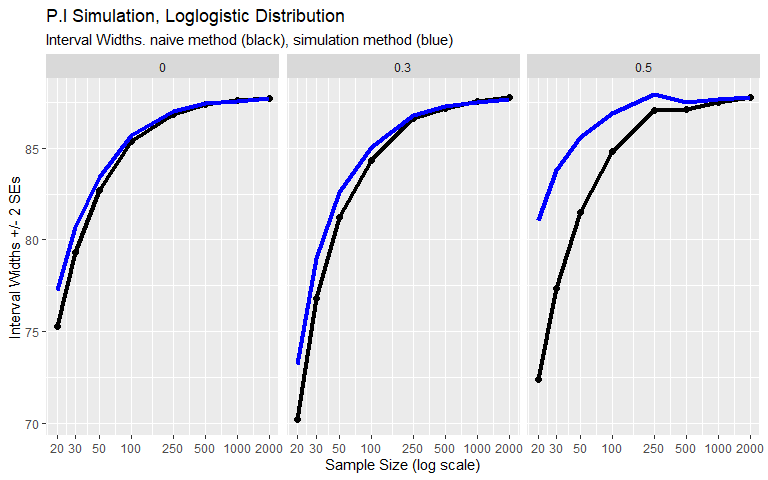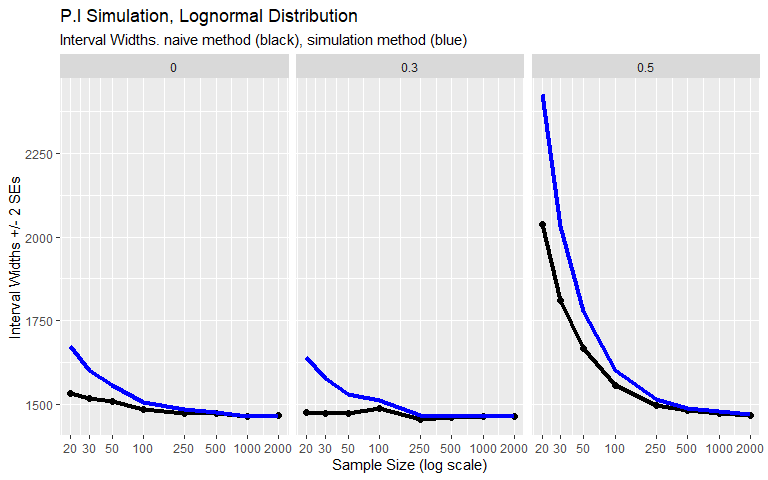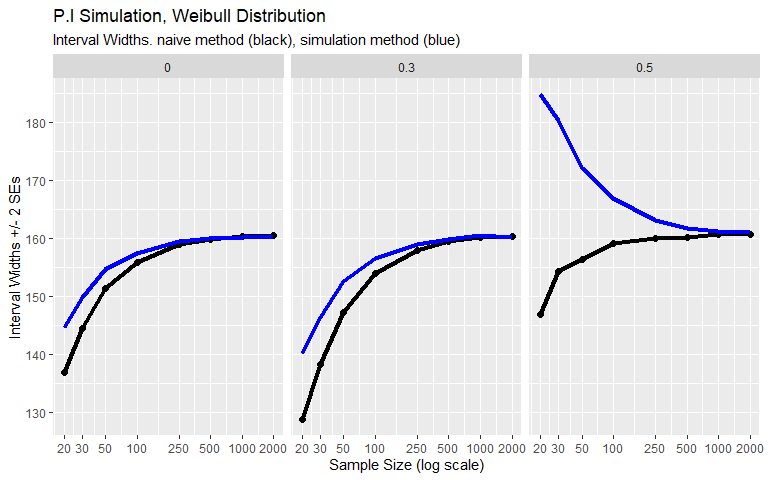References:

Meeker, William Q., and Luis A. Escobar. Statistical methods for reliability data. John Wiley & Sons, 2014. (Chapter 4, 8, and Appendix B)

Harrell, Frank E. Regression modeling strategies. Springer, 2015. (Chapter 17)

sessionInfo()
## R version 4.0.0 (2020-04-24)
## Platform: x86_64-w64-mingw32/x64 (64-bit)
## Running under: Windows 10 x64 (build 17763)
##
## Matrix products: default
##
## locale:
##  LC_COLLATE=C
##  LC_CTYPE=English_United States.1252
##  LC_MONETARY=English_United States.1252
##  LC_NUMERIC=C
##  LC_TIME=English_United States.1252
##
## attached base packages:
##  stats     graphics  grDevices utils     datasets  methods   base
##
## other attached packages:
##   SPREDA_1.1      nlme_3.1-147    survival_3.1-12 here_0.1
##   arm_1.11-1      lme4_1.1-23     Matrix_1.2-18   MASS_7.3-51.5
##   knitr_1.28      broom_0.5.6     ciTools_0.5.2   forcats_0.5.0
##  stringr_1.4.0   dplyr_1.0.0     purrr_0.3.4     readr_1.3.1
##  tidyr_1.0.2     tibble_3.0.1    ggplot2_3.3.0   tidyverse_1.3.0
##
## loaded via a namespace (and not attached):
##   httr_1.4.1       jsonlite_1.6.1   splines_4.0.0    modelr_0.1.7
##   assertthat_0.2.1 statmod_1.4.34   highr_0.8        cellranger_1.1.0
##   yaml_2.2.1       pillar_1.4.3     backports_1.1.6  lattice_0.20-41
##  glue_1.4.1       digest_0.6.25    rvest_0.3.5      minqa_1.2.4
##  colorspace_1.4-1 htmltools_0.4.0  pkgconfig_2.0.3  haven_2.2.0
##  scales_1.1.0     generics_0.0.2   farver_2.0.3     ellipsis_0.3.0
##  withr_2.2.0      cli_2.0.2        magrittr_1.5     crayon_1.3.4
##  readxl_1.3.1     evaluate_0.14    fs_1.4.1         fansi_0.4.1
##  xml2_1.3.2       tools_4.0.0      hms_0.5.3        lifecycle_0.2.0
##  munsell_0.5.0    reprex_0.3.0     compiler_4.0.0   rlang_0.4.6
##  grid_4.0.0       nloptr_1.2.2.1   rstudioapi_0.11  labeling_0.3
##  rmarkdown_2.1    boot_1.3-24      codetools_0.2-16 gtable_0.3.0
##  abind_1.4-5      DBI_1.1.0        R6_2.4.1         lubridate_1.7.8
##  utf8_1.1.4       rprojroot_1.3-2  stringi_1.4.6    Rcpp_1.0.4.6
##  vctrs_0.3.0      dbplyr_1.4.3     tidyselect_1.1.0 xfun_0.13
##  coda_0.19-3